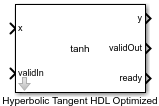Main Content

# Hyperbolic Tangent HDL Optimized

Computes CORDIC-based hyperbolic tangent and generates optimized HDL code

• Library:
• Fixed-Point Designer HDL Support / Math Operations

•## Description

The Hyperbolic Tangent HDL Optimized block returns the hyperbolic tangent of x, computed using a CORDIC-based implementation optimized for HDL code generation.

## Ports

### Input

expand all

Angle in radians, specified as a real finite scalar. If x is a fixed-point or scaled double data type, x must use binary-point scaling. Slope-bias representation is not supported for fixed-point data types.

Data Types: `single` | `double` | `fixed point`

Whether input is valid, specified as a Boolean scalar. This control signal indicates when the data from the x input port is valid. When this value is `1` (`true`), the block captures the value on the x input port. When this value is `0` (`false`), the block ignores the input samples.

Data Types: `Boolean`

### Output

expand all

Hyperbolic tangent of the value at x, returned as a scalar. The value at y is the CORDIC-based approximation of the hyperbolic tangent of x. When the input to the function is floating point, the output data type is the same as the input data type. When the input is a fixed-point data type, the output has the same word length as the input and a fraction length equal to 2 less than the word length.

Data Types: `single` | `double` | `fixed point`

Whether the output data is valid, returned as a Boolean scalar. When the value of this control signal is `1` (`true`), the block has successfully computed the output y. When this value is `0` (`false`), the output data is not valid.

Data Types: `Boolean`

Whether the block is ready, returned as a Boolean scalar. This control signal indicates when the block is ready for new input data. When this value is 1 (`true`), and the validIn value is 1 (`true`), the block accepts input data in the next time step. When this value is 0 (`false`), the block ignores input data in the next time step.

Data Types: `Boolean`

expand all

## See Also

### Functions

Introduced in R2020a

## SupportGet trial now